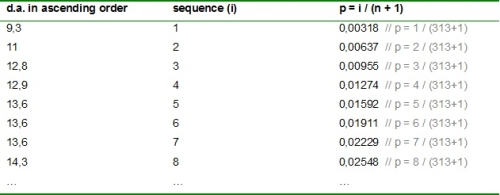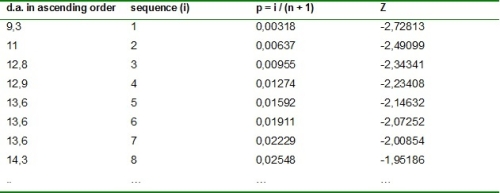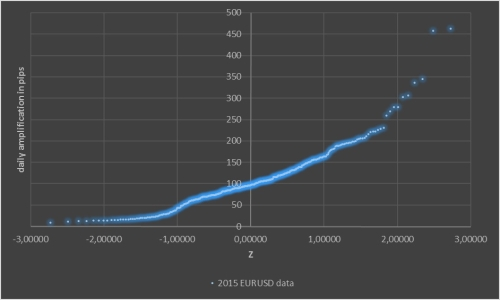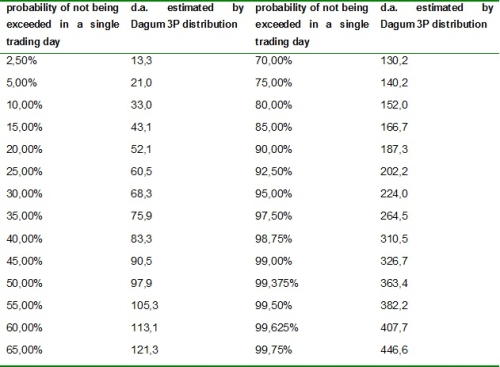2015’s EURUSD daily amplification in pips (also shortly written in this article as d.a.) study has been done to ask offer side values.

For the year of 2015 there are 313 trading days that will be carried into observation (data gathered in Dukascopy website ).
Chart 1 shows all the mentioned data (in ‘yy’ d.a. and in ‘xx’ the number of observed trading days).

Chart 1 – EURUSD d.a. in 2015After chart 1 analysis, it’s hard to figure out a pattern for EURUSD d.a. in 2015 – in order to better understand the data placed in the previous chart, it’s essential to reshape data – the operations carried on are carefully mentioned in the following text:
1. sort all EURUSD d.a. values in ascending order, as shown in table 1;

Table 1 – Sorting in ascending order EURUSD d.a. values2. for each sorted EURUSD d.a. values, enumerate them in ascending order with an integer number starting by number 1, as shown in table 2;

Table 2 – Enumerating in ascending order sorted EURUSD d.a. values3. attribute for each sorted EURUSD d.a. values a probability of not being exceeded in a single trading day given by the following expression:
• p = i / (n + 1);
• p ≡ d.a. probability of not being exceeded in a single trading day;
• i ≡ enumerated integer number for each sorted d.a. value;
• n ≡ number of observed trading days – 313 in 2015;
as shown in table 3;

Table 3 – Attributing a probability for each EURUSD d.a. value of not being exceeded in a single trading day4. for each obtained probability calculate the respective standard normal Z value, as shown in table 4;

Table 4 – Calculating standard normal Z valuesAfter reaching all these operations we have conditions to display a new chart, in ‘yy’ sorted in ascending order EURUSD d.a. and in ‘xx’ standard normal Z values, as shown in chart 2.

Chart 2 – Sorted in ascending order EURUSD d.a. in 2015After chart 2 analysis, we verify a pattern.
In order to represent the data in chart 2 it was added a statistical distribution, a Dagum 3P (3 parameters) as shown in chart 3.

Chart 3 – Sorted in ascending order EURUSD d.a. in 2015 & Dagum 3P distributionTable 5 resumes Dagum 3P estimated values placed in chart 3. It matches the probabilities and estimated EURUSD d.a. values of not being exceeded in a single trading day.

Table 5 – Dagum 3P estimated d.a. values and each probability of not being exceeded in a single tradingThe values obtained in table 5 can be used as reference values, which are linked with a probability of not being exceeded in a single trading day for EURUSD in 2015.
It can help traders this year to figure out daily momentums regarding 2015’s EURUSD estimated reference values.
Dagum 3P estimated values placed in chart 3 and resumed in table 5 for EURUSD in 2015 gives a:
• d.a. lower than 60,5 pips in 25% of trading days;
• d.a. lower than 97,9 pips in 50% of trading days;
• d.a. lower than 140,2 pips in 75% of trading days;
• d.a. lower than 224,0 pips in 95% of trading days;
• d.a. lower than 326,7 pips in 99% of trading days;
• d.a. lower than 446,6 pips in 99,75% of trading days.

 https://www.dukascopy.com/swiss/english/marketwatch/historical/
Translate to English Show original